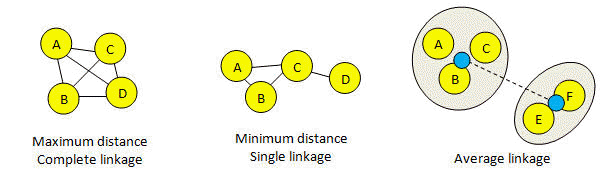In agglomerative clustering, linkage specifies how the distance between two clusters is calculated. If the clustering is used to construct a tree, linkage determines the order internal nodes are created and hence the tree topology. If the clustering is used to find groups at a given distance threshold, linkage determines where the final cluster boundaries occur. In USEARCH, linkage is specified using the -linkage option.

USEARCH supports three types of linkage in which the distance between two clusters is calculated by considering pairs of sequences (one from each cluster), and taking the maximum, minimum or average distance over all pairs.

If the final clusters are determined by a fixed distance threshold, e.g. 97% identity, then maximum and minimum linkage are equivalent to complete and single linkage, respectively.

Complete linkage means that all pairs of sequences in a cluster must be closer than the threshold. This means that clusters tend to be smaller compared to single or average linkage, because a new sequence must be close enough to all existing sequences, not just one as in the case of single linkage. With complete linkage, the diameter of the cluster  (the maximum distance between a pair) cannot be larger than the distance threshold. Complete linkage is equivalent to maximum linkage and to finding the cliques of a graph where the edges include all pair-wise distances within the threshold.

Single linkage means that a sequence should be included in a cluster if the distance to any other sequence is below the threshold. This means that clusters tend to grow large as new sequences are introduced, because a new sequence only needs to be close to one existing sequence inside the cluster. This means that with single linkage there is no upper bound on the diameter of a cluster. Single linkage is equivalent to minimum linkage and to finding the connected components of a graph where the edges include all pair-wise distances within the threshold.

Average linkage is harder to visualize. In the figure below, black lines represent distances below the threshold. The blue dots in the figure for average linkage represent "average sequences" for the two existing clusters {ABC} and {EF}, and the dotted line represents the distance between them. The clusters are joined if this distance is less than the threshold. This picture is designed to give an intuitive idea of how clusters are created, but it should not be taken too seriously. In fact, the average is calculated over all pairs. In this example, the average is (AE + AF + BE + BF + CE + CF)/6, where XY means the distance between sequences X and Y. With average linkage, clusters tend to be larger than maximum linkage and smaller than minimum linkage. The diameter of a cluster can be larger than the threshold.

Using average linkage is equivalent to the UPGMA algorithm that was introduced for making OTUs in classical numerical taxonomy before the development of phylogenetic tree reconstruction methods such as Neighbor-Joining and next-generation clustering algorithms such as UPARSE. Today, UPGMA should be considered obsolete for OTU clustering of biological sequences.